# The superconductivity theory of Ginzburg-Landau

01/01/1950

In physics, Ginzburg–Landau theory, often called Landau–Ginzburg theory, named after Vitaly Lazarevich Ginzburg and Lev Landau, is a mathematical physical theory used to describe superconductivity. In its initial form, it was postulated as a phenomenological model which could describe type-I superconductors without examining their microscopic properties. Later, a version of Ginzburg–Landau theory was derived from the Bardeen–Cooper–Schrieffer microscopic theory by Lev Gor'kov, thus showing that it also appears in some limit of microscopic theory and giving microscopic interpretation of all its parameters.

Based on Landau's previously-established theory of second-order phase transitions, Ginzburg and Landau argued that the free energy, F, of a superconductor near the superconducting transition can be expressed in terms of a complex order parameter field, ψ, which is nonzero below a phase transition into a superconducting state and is related to the density of the superconducting component, although no direct interpretation of this parameter was given in the original paper. Assuming smallness of |ψ| and smallness of its gradients, the free energy has the form of a field theory.

where Fn is the free energy in the normal phase, α and β in the initial argument were treated as phenomenological parameters, m is an effective mass, e is the charge of an electron, A is the magnetic vector potential, and B = ∇ x A is the magnetic field. By minimizing the free energy with respect to variations in the order parameter and the vector potential, one arrives at the Ginzburg–Landau equations

where j denotes the dissipation-less electric current density and Re the real part. The first equation — which bears some similarities to the time-independent Schrödinger equation, but is principally different due to a nonlinear term — determines the order parameter, ψ. The second equation then provides the superconducting current.

Consider a homogeneous superconductor where there is no superconducting current and the equation for ψ simplifies to:

### α ψ + β|ψ|2 ψ = 0

This equation has a trivial solution: ψ = 0. This corresponds to the normal state of the superconductor, that is for temperatures above the superconducting transition temperature, T > Tc.

Below the superconducting transition temperature, the above equation is expected to have a non-trivial solution (that is ψ ≠ 0). Under this assumption the equation above can be rearranged into:

### |ψ|2 = - α/β

When the right hand side of this equation is positive, there is a nonzero solution for ψ (remember that the magnitude of a complex number can be positive or zero). This can be achieved by assuming the following temperature dependence of α: α(T) = α0 (T − Tc) with α0/β > 0:

Above the superconducting transition temperature, T > Tc, the expression α(T)/β is positive and the right hand side of the equation above is negative. The magnitude of a complex number must be a non-negative number, so only ψ = 0 solves the Ginzburg–Landau equation.

Below the superconducting transition temperature, T < Tc, the right hand side of the equation above is positive and there is a non-trivial solution for ψ. Furthermore,

### |ψ|2 = - α0(T – Tc)/β

that is ψ approaches zero as T gets closer to Tc from below. Such a behaviour is typical for a second order phase transition.

In Ginzburg–Landau theory the electrons that contribute to superconductivity were proposed to form a superfluid. In this interpretation, |ψ|2 indicates the fraction of electrons that have condensed into a superfluid.

The Ginzburg–Landau equations predicted two new characteristic lengths in a superconductor which was termed coherence length, ξ. For T > Tc (normal phase), it is given by

while for T < Tc (superconducting phase), where it is more relevant, it is given by

It sets the exponential law according to which small perturbations of density of superconducting electrons recover their equilibrium value ψ0. Thus this theory characterized all superconductors by two length scales. The second one is the penetration depth, λ. It was previously introduced by the London brothers in their London theory. Expressed in terms of the parameters of Ginzburg–Landau model it is

where ψ0 is the equilibrium value of the order parameter in the absence of an electromagnetic field. The penetration depth sets the exponential law according to which an external magnetic field decays inside the superconductor.

The original idea on the parameter "k" belongs to Landau. The ratio κ = λ/ξ is presently known as the Ginzburg–Landau parameter. It has been proposed by Landau that Type I superconductors are those with 0 < κ < 1/√2, and Type II superconductors those with κ > 1/√2.

Taking into account fluctuations. For Type II superconductors, the phase transition from the normal state is of second order, as demonstrated by Dasgupta and Halperin. While for Type I superconductors it is of first order as demonstrated by Halperin, Lubensky and Ma.

#### Classification of superconductors based on Ginzburg–Landau theory

In the original paper Ginzburg and Landau observed the existence of two types of superconductors depending on the energy of the interface between the normal and superconducting states.The Meissner state breaks down when the applied magnetic field is too large. Superconductors can be divided into two classes according to how this breakdown occurs. In Type I superconductors, superconductivity is abruptly destroyed when the strength of the applied field rises above a critical value Hc. Depending on the geometry of the sample, one may obtain an intermediate state consisting of a baroque pattern of regions of normal material carrying a magnetic field mixed with regions of superconducting material containing no field. In Type II superconductors, raising the applied field past a critical value Hc1 leads to a mixed state (also known as the vortex state) in which an increasing amount of magnetic flux penetrates the material, but there remains no resistance to the flow of electric current as long as the current is not too large. At a second critical field strength Hc2, superconductivity is destroyed. The mixed state is actually caused by vortices in the electronic superfluid, sometimes called fluxons because the flux carried by these vortices is quantized. Most pure elemental superconductors, except niobium and carbon nanotubes, are Type I, while almost all impure and compound superconductors are Type II.

The most important finding from Ginzburg–Landau theory was made by Alexei Abrikosov in 1957. He used Ginzburg–Landau theory to explain experiments on superconducting alloys and thin films. He found that in a type-II superconductor in a high magnetic field, the field penetrates in a triangular lattice of quantized tubes of flux vortices.

#### Landau–Ginzburg theories in string theory

In particle physics, any quantum field theory with a unique classical vacuum state and a potential energy with a degenerate critical point is called a Landau–Ginzburg theory. The generalization to N = (2,2) supersymmetric theories in 2 spacetime dimensions was proposed by Cumrun Vafa and Nicholas Warner in the November 1988 article Catastrophes and the Classification of Conformal Theories, in this generalization one imposes that the superpotential possess a degenerate critical point. The same month, together with Brian Greene they argued that these theories are related by a renormalization group flow to sigma models on Calabi–Yau manifolds in the paper Calabi–Yau Manifolds and Renormalization Group Flows. In his 1993 paper Phases of N = 2 theories in two-dimensions, Edward Witten argued that Landau–Ginzburg theories and sigma models on Calabi–Yau manifolds are different phases of the same theory. A construction of such a duality was given by relating the Gromov–Witten theory of Calabi–Yau orbifolds to FJRW theory an analogous Landau–Ginzburg "FJRW" theory in The Witten Equation, Mirror Symmetry and Quantum Singularity Theory. Witten's sigma models were later used to describe the low energy dynamics of 4-dimensional gauge theories with monopoles as well as brane constructions. Gaiotto, Gukov & Seiberg (2013)Ginzburg Landau Theory, Coherence length and penetration depthSuperconduction - Ginzburg Landau theoryMod-01 Lec-29 Ginsburg - Landau Theory, Flux QuantizationSuperconductivity: Ginzburg-Landau TheoryOn the Supercondutivity Theory - Paper translated from Russian

REFERENCES

California Institute of Technology. Available in: http://www.cmp.caltech.edu/~mcc/Ph127/c/Lecture14.pdf. Access in: 20/10/2018.

University of Florida. Available in: http://www.phys.ufl.edu/~pjh/teaching/phz7427/7427notes/ch5.pdf. Access in> 20/10/2018.

Wikipedia. Available in: https://en.wikipedia.org/wiki/Ginzburg%E2%80%93Landau_theory. Access in: 20/10/2018.

Comment

Author

Info

Published in 21/10/2018

Updated in 19/02/2021

All events in the topic Condensed Matter Physics:

01/01/1820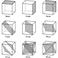Classification of crystalline symmetries
01/01/1879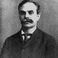Hall Effect
01/01/190001/01/1905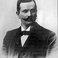Drude and Lorentz model on electric conduction
08/04/1911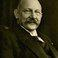Discovery of mercury superconductivity by Onnes
01/10/1913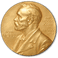H. K. Onnes receives the Nobel Prize
10/10/1914Max von Laue receives the Nobel Prize
01/10/1915Sir W. H. Bragg and W. L. Bragg share Nobel Prize
01/10/1930Sir C. V. Raman receives the Nobel Prize
01/10/1956Shockley, Bardeen and Brattain share Nobel Prize
01/10/1962L. D. Landau receives the Nobel Prize
01/10/1972Bardeen, Cooper and Schrieffer share the Nobel Prize
23/06/1913Study of Crystals using X-rays by W.H. & W.L. Bragg
01/01/1912Discovery of X-ray diffraction by Crystals by Van Laue
21/02/1928Raman scattering
01/01/192801/01/1933Quantum Theory in Solids
16/12/1947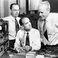Transistor Effect
01/01/1950The superconductivity theory of Ginzburg-Landau
18/02/1957Theory of Superconductivity BCS
08/06/1962Josephson Effect tunneling in superconductors
01/01/1965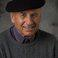Density Functional Theory
01/01/1971Superfluid helium-3
01/01/1973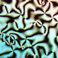Liquid Crystal Theory
10/10/198001/10/1982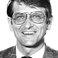Integer and Fractional Quantum Hall Effect
10/10/1982Discovery of Quasi-crystals
11/09/1985Fullerene 60
01/10/1986High-temperature superconductivity
01/01/1988Giant magnetoresistance
01/06/1991Carbon nanotube
01/01/2004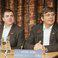Discovering Graphene
08/12/2017Excitonium